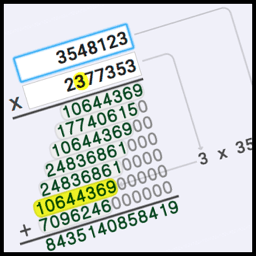# I Got Skills... They're Multiplying!We spend a lot of time focused on multiplication tables, and getting past the stages of memorizing math facts is an important milestone. By the time we are working with multi-digit problems, we're starting to deal with algorithms, the series of steps, necessary to solve problems. Fortunately, learning multi-digit addition and multi-digit subtraction, even with regrouping, is usually achieved without too much difficulty. The steps for multi-digit multiplication require are a bit more demanding... In fact, it has all the fun of multi-digit addition on steriods right along with the twists multiplication brings as well. However, I've got a great tool that should take away some of the long multiplication anxiety... Visual Multiplication Calculator This calculator will let you multiply two long numbers together, and it shows you the intermediate products as it goes. Put a couple of numbers in there and hover your mouse over the work produced by the calculator... You'll see what parts from the multiplicand and the multiplier are used to create each step, and it clearly shows how the place values are preserved as we work through the multiplier digits. It's a great tool for developing an understanding of the conventional steps for multi-digit multiplication. But, the most important thing is practice! These multi-digit multiplication worksheets work hand-in-hand with the multiplication calculator. If you need review, be sure to also check out the complete series of multiplication worksheets, multiplication tables, and multiplication charts to really get your multiplication facts polished up!